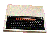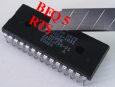×   Main Menu ALL The 8BS News Manuals (New menu) Links Worth a Look Tools Disc and Basic Webring Site Map 8BS Guestbook Old Guest Book Me The Barnsley Rovers# The BBC and Master Computer Public Domain Library

### A59B TAN

Submitted by Steve Fewell

Description:

This routine calls &A93A to obtain the Floating-point argument and use continued-fraction expressions to calculate
either the Sine or Cosine value (depending on the value of the argument) of the argument.
This FWA result is stored in temporary Floating-point location &047B-&047F.

Call &A917 (SIN) to calculate the Sine value from the FWA result.
Store the Sine result in the temporary Floating-point location &0476-&047A.

Set the FWA back to the &A93A result (from the Temporary Floating-point variable at &047B-&047F).
Call &A915 (COS) to calculate the Cosine value from the &A93A result.
Note: Storing the &A93A value avoids the need to calculate it again!

Set argp to point to &0476 (the Sine result).
Call &A5EE to divide argp by the FWA [FWA = argp / FWA], where argp points to the Sine result and the FWA contains
the Cosine result.
Dividing the Sine result by the Cosine result gives the Tangent value.

Exit with A = #&FF (as the result is Floating-point and is located in the FWA).

Example 1: TAN(90)
Divide the SIN(90) result by the COS(90) result
FWA = 0.8939945 / -0.4480777 --> -1.9951774 (= the TAN result)

Example 2: TAN(2.41)
Divide the SIN(2.41) result by the COS(2.41) result
FWA = 0.6680554 / -0.7441115 --> -0.8977893 (= the TAN result)

Example 3: TAN(1.5)
Divide the SIN(1.5) result by the COS(1.5) result
FWA = 0.9974949 / 0.0707372 --> 14.1014191 (= the TAN result)

Example 4: TAN(5.63)
Divide the SIN(5.63) result by the COS(5.63) result
FWA = -0.6078147 / 0.7940788 --> -0.7654337 (= the TAN result)

Example 5: TAN(0)
Divide the SIN(0) result by the COS(0) result
FWA = 0 / 1 --> 0 (= the TAN result)

Example 6: TAN(-0.75)
Divide the SIN(-0.75) result by the COS(-0.75) result
FWA = -0.6816387 / 0.7316889 --> -0.9315963 (= the TAN result)

Example 7: TAN(0.25)
Divide the SIN(0.25) result by the COS(0.25) result
FWA = 0.2474039 / 0.9689124 --> 0.2553418 (= the TAN result)

This diagram shows the relationship between the trig functions.

### Disassembly for the TAN routine

 A59B : 032 058 169 20 3A A9 JSR &A93A Get value and calculate Sine/Cosine value A59E { 169 123 A9 7B LDA#&7B A5A0 032 019 165 20 13 A5 JSR &A513 Store FWA to &047B A5A3 032 023 169 20 17 A9 JSR &A917 SIN (convert the result of &A93A to the Sine result) A5A6 v 169 118 A9 76 LDA#&76 A5A8 032 019 165 20 13 A5 JSR &A513 Store FWA to &047B A5AB { 169 123 A9 7B LDA#&7B A5AD ; 032 059 165 20 3B A5 JSR &A53B Load FWA from variable at &0400 + A (i.e. &047B) A5B0 032 021 169 20 15 A9 JSR &A915 COS (convert the result of &A93A to the Cosine result) A5B3 v 169 118 A9 76 LDA#&76 A5B5 032 148 165 20 94 A5 JSR &A594 Set argp to &0400 + A (i.e. &0476) A5B8 032 238 165 20 EE A5 JSR &A5EE Floating-Point Division [FWA=argp/FWA] A5BB 169 255 A9 FF LDA#&FF A5BD ` 096 60 RTSOr